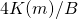### Never twice the same

“Ever present, never twice the same;
Ever changing, never less than whole”~Robert Irwin

If for an instant he dozed off, the image of Fantômas took shape in his mind, but never twice the same: sometimes he saw a colossal figure with bestial face and muscular shoulders; sometimes a wan, thin creature, with strange and piercing eyes; sometimes a vague form, a phantom—Fantômas!Marcel Allain, Pierre Souvestre, Fantomas

In the last post, Taming the T-handle continued, we have learned how to model free rotation of an asymmetric rigid body in that very-very special case when the ratio of the angular momentum squared to the kinetic energy doubled is exactly– the middle moment of inertia. In that case only one flip of the axis happens. For an infinite time, in the past, and in the future, the T-handle rotates along theaxis, or rather: very close to this axis. In its whole infinitely long life only once it flips, in our case nearI say “near”, since what is, say, when compared with infinity?!

Of course in practice we will never be able to achieve this particular ratio of angular momentum and kinetic energy exactly! But who cares? In practice there are no perfect straight lines and there are no perfect spheres. So what? When the ratio is “slightly” different, when the parameterthat we used in all previous posts,is “slightly” different from, then the motion is quasi-periodic, though I am not sure if it is indeed quasi-periodic according to the mathematical definition of quasiperiodicity. From Wikipedia:

In mathematics, a quasiperiodic function is a function that has a certain similarity to a periodic function. A functionis quasiperiodic with quasiperiodifwhereis a “simpler” function than. What it means to be “simpler” is vague.

In our case there are flips occurring periodically, the pattern repeats itself is a very similar way (though not exactly the same) again and again, the period can be calculated using elliptic-function. We have discussed it before, though we did not stress this phenomenon of “quasi” periodicity.

In Taming the T-handle continued we have derived several important formulas. We have

(1)where

(2)(3)and(for) is given by:is periodic, with periodButis not periodic at all. The functionsandinare periodic, butis a sum of two terms. The first term, linear in, would makeperiodic. with period, but the second term spoils any periodicity whatsoever. That is why the flips, though governed by the periodicityfrom the elliptic functionsnever repeat exactly the same way.In a comment to the last past Bjab formulated the following hypothesis:

So now there is a hypothesis that:
velocity of the end of the leg of T-handle is always perpendicular to the plane of T-handle.
Always means: when m=1

Proving (or disproving) this hypothesis is a good exercise. My T-handle is defined by the following Mathematica code:In fact I have two slightly shifted T-handles, of different colors, so that it looks like one but painted top red, bottom yellow. The shift is in-direction, so that my T-handle is all in the planeThe leg is along theaxis, from -0.9 to 0.2. We can assume that the leg end is atThe pointmoves with time along the trajectory:The plane of the T-handle is also rotated by the matrixtherefore the angle between the velocity vector and the plane is the same as the angle betweenand theplane. ButThushas components. This vector is orthogonal tobut is at the angletoTherefore it seems that the hypothesis, though fruitful and interesting, should not be considered as proven.
Update: Why? We know thatThusWe want to find the angle, so we are interested in normalized vector. Therefore we can skip the commonfactor. Thus we see that the angle is constant in time – which is an interesting fact. In particular forwe haveThe square root of the sum of squares isTherefore the normalized first component isWe have